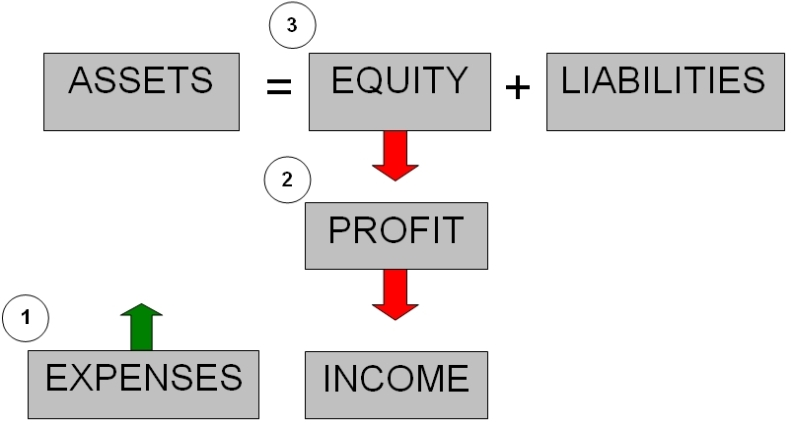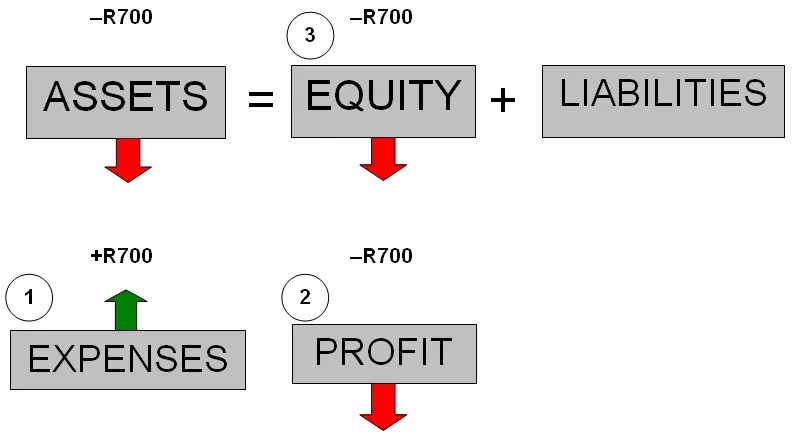# Supplies Expense Example: Accounting Equation & Journal EntryQ: How do you account for "estimated expenses" in the accounting equation?

For example: Estimated supplies used for 6 months R700.

(R = Rands = South African currency)

How will this transaction affect the accounting equation?

A:
Regardless of the accounting equation or anything else, estimated expenses is unusual. All expenses should be tracked and calculated accurately without any need to "estimate" them.

However, the situation might arise where a certain expense was not closely tracked and so a reasonable estimate would serve as the next best thing.

An estimated expense, supplies expense or any other, is still an
expense. So the question is really "how do you account for the supplies expense in the accounting equation?"

Expenses (1) are the opposite of income. Expenses reduced profit (2), which means less for the owner, so less share of the assets for the owner - less owner's equity (3).Additionally, those supplies that were used are
assets. So when they are used, the assets would decrease:In case you were wondering, the journal entry for the above would be:

DR Supplies Used (expense)...............R700
CR Supplies (asset).........................................R700

Since "Supplies Used" is an expense, and expenses always occurs on the left side, this account is
debited.

"Supplies" is an asset account which is decreasing. Assets increase on the left side (debit) and decrease on the right (credit), so supplies is
credited.

FYI, another term commonly used for supplies that one consumes or uses in ones business is "consumables."

Does that supplies expense example for the accounting equation make sense? Let me know by clicking "Click here to post comments" further below.

And check out the related tutorials and questions just below.

Best,
Michael Celender
Founder of Accounting Basics for Students

Related Questions & Tutorials:

Search this Site: